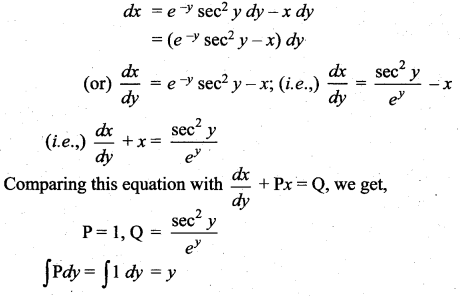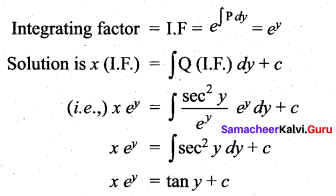Tamilnadu Samacheer Kalvi 12th Maths Solutions Chapter 10 Ordinary Differential Equations Ex 10.7

Solve the following Linear differential equations:

Question 1.Solution: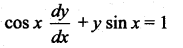Dividing throughout by ‘cos x’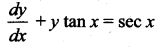This is a linear differential equation of the type $$\frac{d y}{d x}$$ + Py = Q
Where P = tan x,
Q = sec x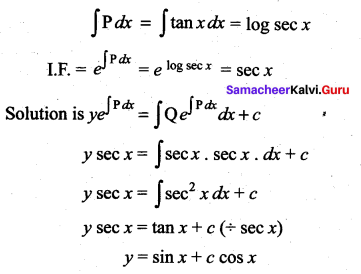Question 2.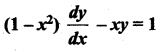Solution: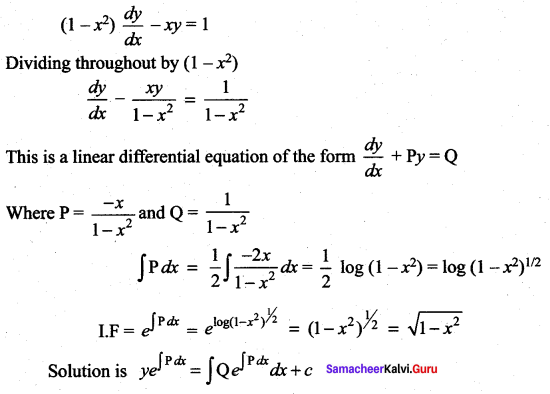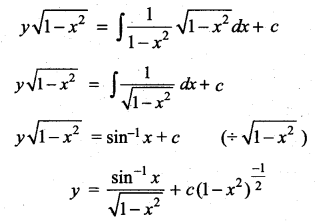Question 3.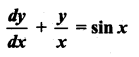Solution: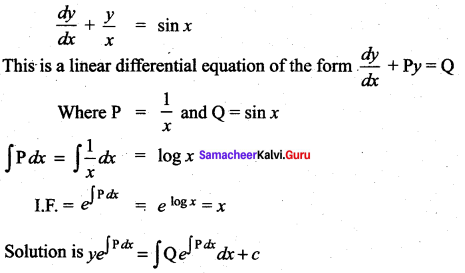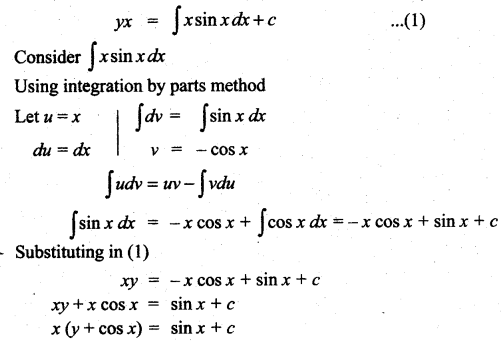Question 4.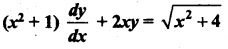Solution: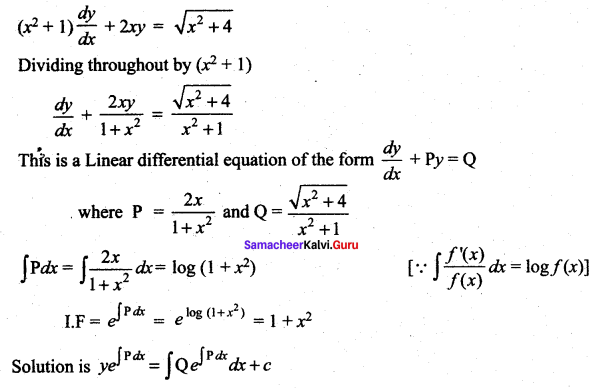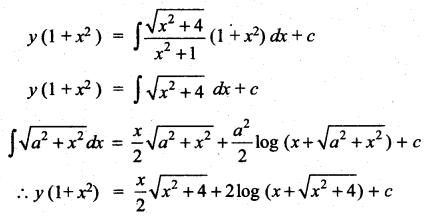Question 5.
(2x – 10y3)dy + ydx = 0
Solution: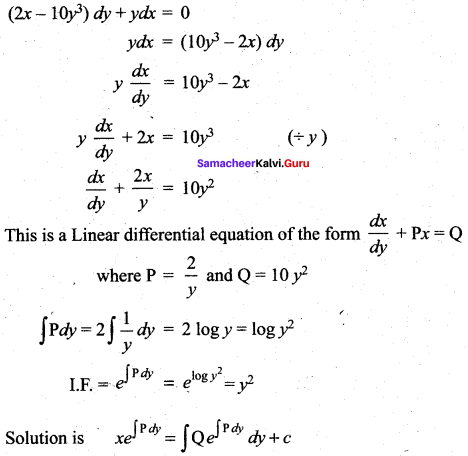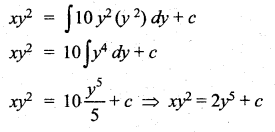Question 6.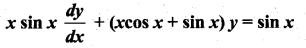Solution: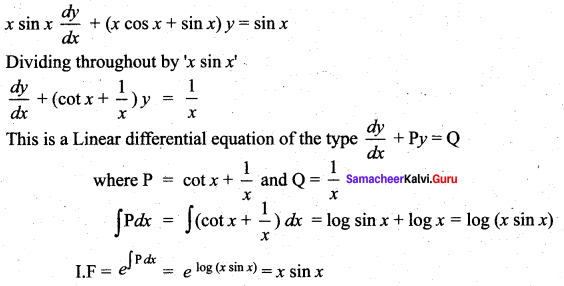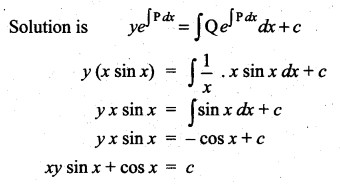Question 7.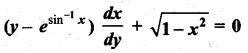Solution: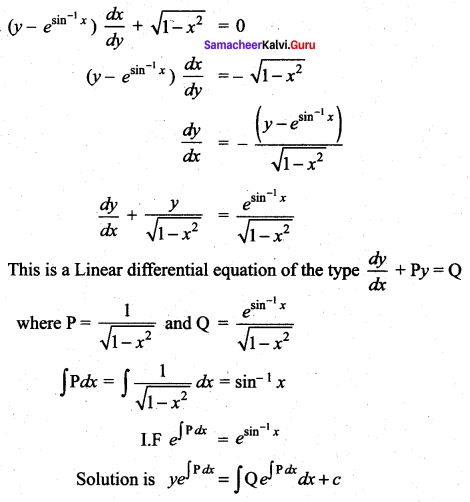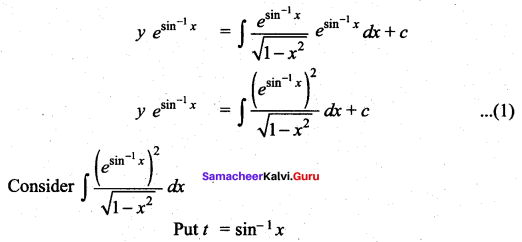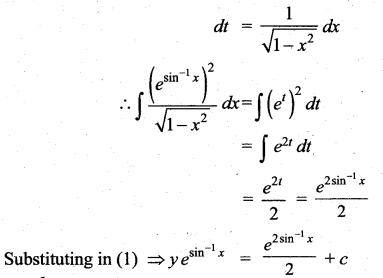Question 8.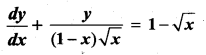Solution: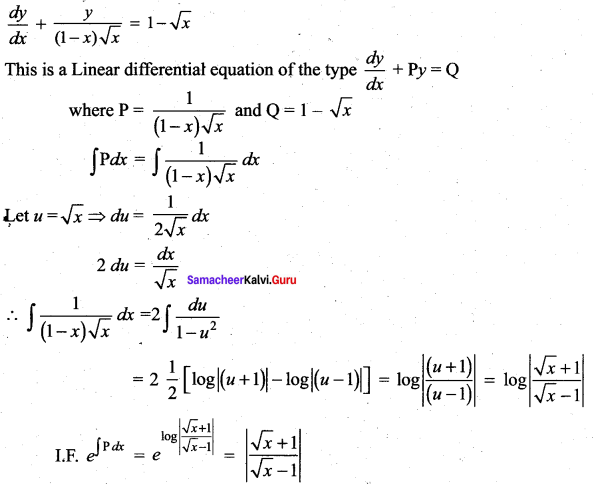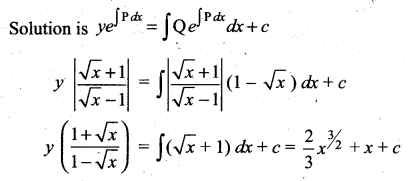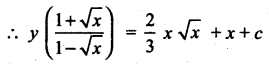Question 9.Solution: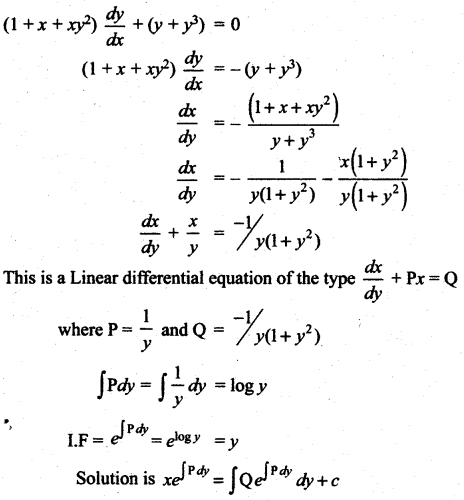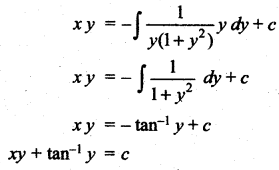Question 10.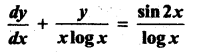Solution: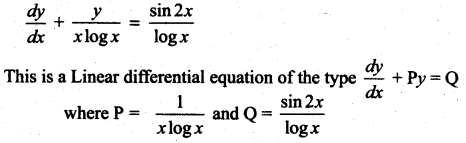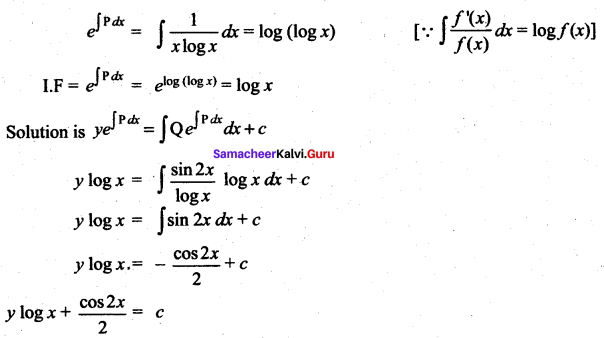Question 11.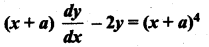Solution: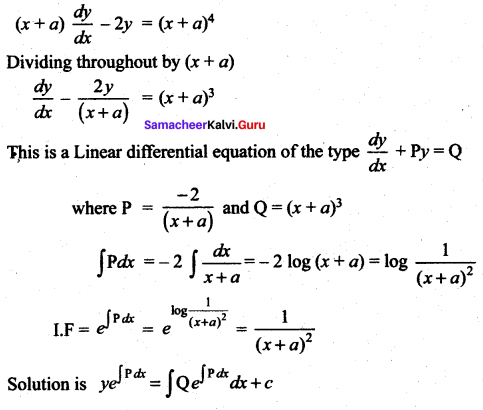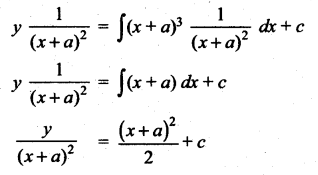2y = (x + a)4 + 2c(x + a)2

Question 12.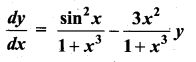Solution: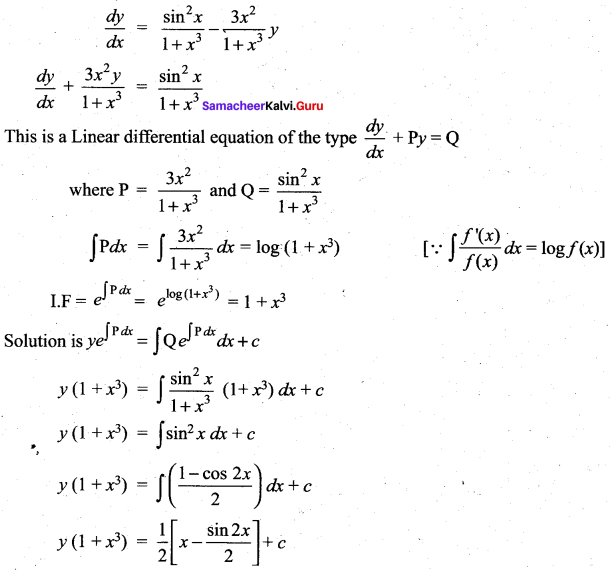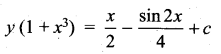Question 13.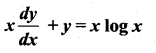Solution: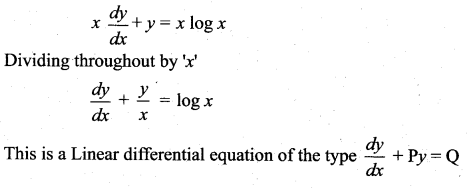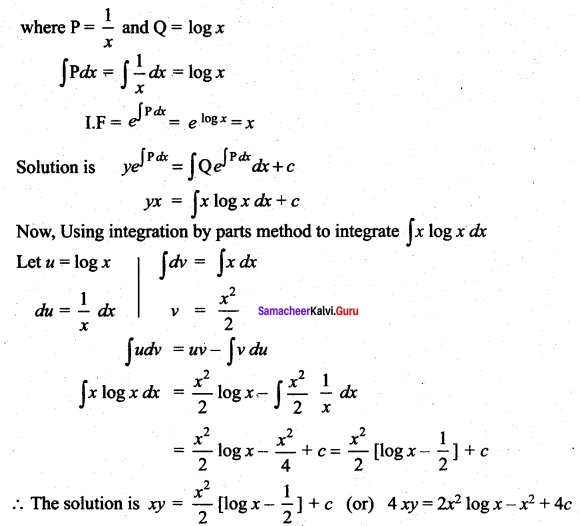Question 14.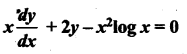Solution: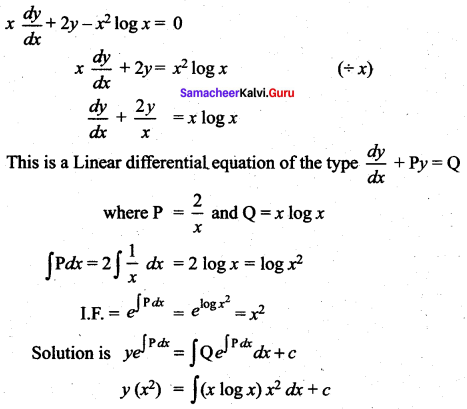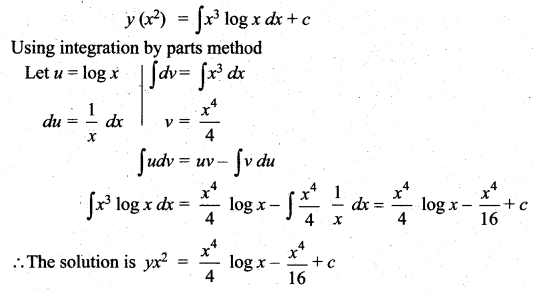Question 15.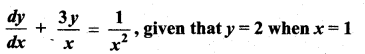Solution: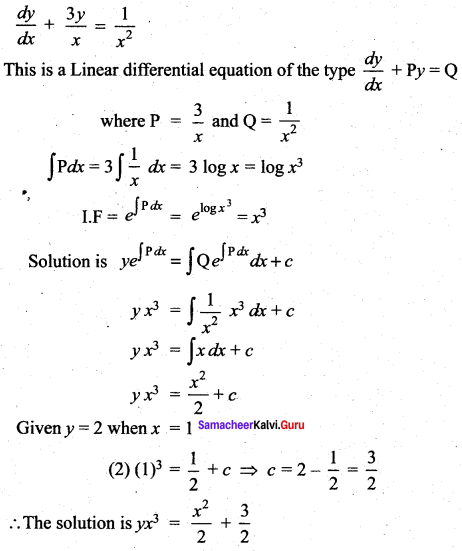2x3y = x2 + 3

Samacheer Kalvi 12th Maths Solutions Chapter 10 Ordinary Differential Equations Ex 10.7 Additional Problems

Question 1.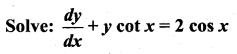Solution: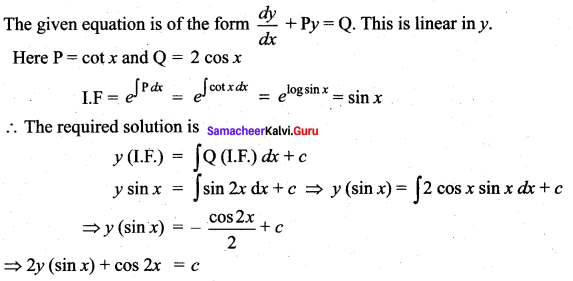Question 2.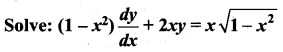Solution: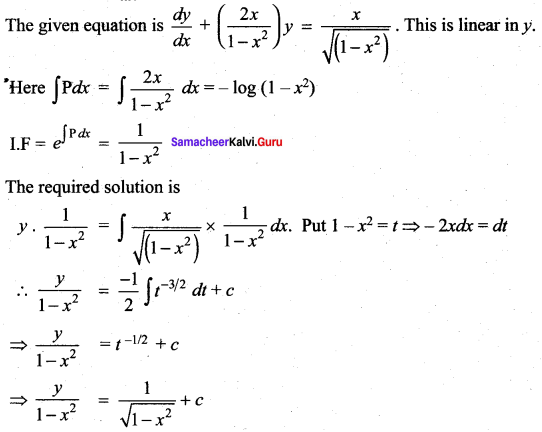Question 3.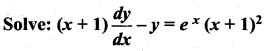Solution: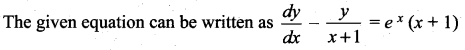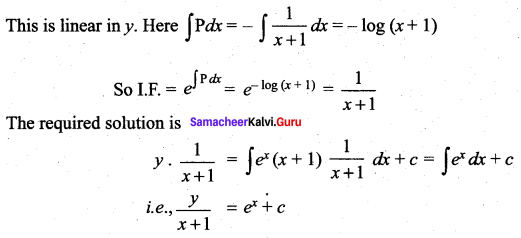Question 4.
Solve: (1 + y2)dx = (tan-1y – x)dy
Solution: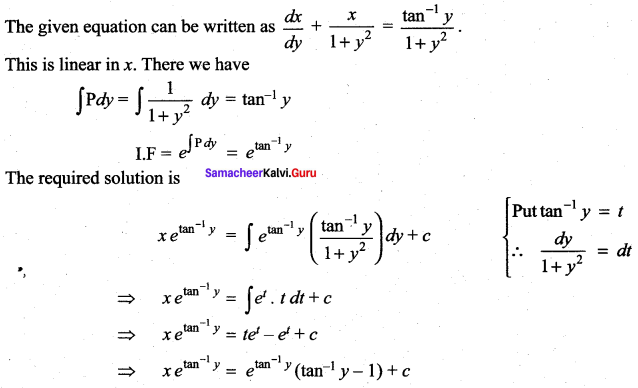Question 5.
Solve: dx + x dy = e-y sec2 y dy
Solution: